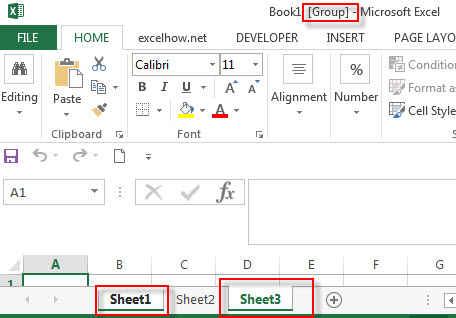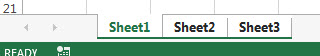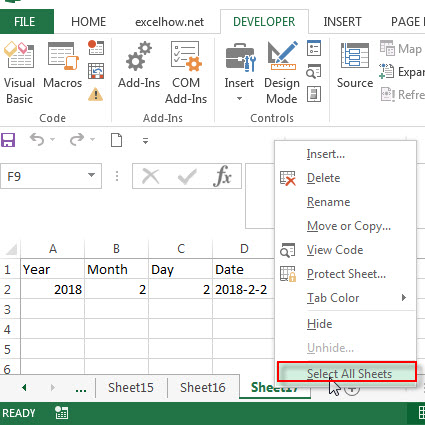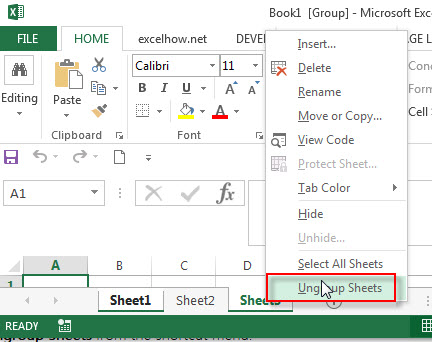# How to group or ungroup sheets in Excel

This post will guide you how to group or ungroup worksheets in your current workbook in excel.

If you want to update one worksheet, then let excel to automatically update others selected worksheets. And there is a useful way to group all of worksheets that you want to update quickly. One of the prerequisites for this method is that the selected worksheet should be have the same structure.

Assuming that you have a large number of worksheets in your workbook, and if you want to perform any operations on only one worksheet, then the other selected worksheets is able to perform the same action at the same time, you need to group these worksheets.

## Grouping worksheets

If you want to group multiple worksheets in a workbook, you can follow the below steps:

1# select one worksheet

2# press Ctrl key to select others worksheets that you want to group3# if you want to group consecutive worksheets, you need to click the first sheet tab that you want to group, and then press and hold down Shift key, then click the last tab of the sheet you want in the group. So all the worksheets between the first and last tab will be grouped.You will see that all the grouped worksheets tabs will turn into white color and the indicator [Group] will appear in the title bar after the name of workbook. It indicated that these selected worksheets have been grouped. And you can perform one operation in sheet1, then the same action will be replicated in each sheet in the same group.

## Grouping all the worksheets in a workbook

If you want to group all the sheets in an active workbook, you can following the below two steps:

1# select one any sheet, then right click on it

2# click “Select All Sheets” from the pop-up menu list.## Ungroup worksheets

If you want to ungroup worksheets, you can follow the below two ways:

Method 1: select one worksheet, right click to grouping worksheet. Then choose Ungroup sheets menu.Method 2: clicking on any worksheet tab in the grouping worksheet.

Related Posts

Cap percentage values between 0 and 100

This article will talk about how to cap the percentage values between 0% and 100% in Microsoft Excel Spreadsheet or Google Sheets. If you are a newbie on Excel or google Sheets, you may be able to do this by ...

Calculate Cap Percentages to Specific Value

This article will talk about how to limit the cap percentage of a given amount to a given value in Microsoft Excel Spreadsheet or Google Sheets. If you are a newbie on Excel or google Sheets, you may be able ...

Calculate Win Loss Tie

Suppose you got a task to calculate the win, loss, and tie totals; what would you do? If you are new to Ms Excel and don't have enough experience with it, then you might do this task manually but let ...

Calculate Years Between Dates In Ms Excel

If you are an avid Ms Excel user, then you might have come across a task in which you needed to calculate the years between the dates; you might take it easy and do this task manually, which is also ...

Calculate Number of Hours between Two Times

Calculating the difference between two times might be a valuable statistic for subsequent computations or averages, whether you're producing a time sheet for staff or recording personal exercises. While Excel has a plethora of complex functions, including date and time ...

Calculate Loan Interest in Given Year

When you borrow money, you are supposed to repay it gradually. Lenders, on the other hand, want to be compensated for their services and the risk they incur by lending you money. That is, you will not just repay the ...

Calculate Interest Rate for Loan

The interest rate is the fee charged by a lender to a borrower and is expressed as a percentage of the principal—the lent amount. The interest rate on a loan is often expressed as an annual percentage rate, abbreviated as ...

Calculate Interest for Given Period

Using the IPMT function in Excel, we can compute the interest payment on any loan. This step-by-step tutorial will guide Excel users of all skill levels through the process to calculate interest for given period. Finally, the formula: =IPMT(B3/12,1,B5,-B2) The ...

How To Use Excel GCD Function

This post will guide you how to use Excel GCD function with syntax and examples in Microsoft excel. Description The Excel GCD function Returns the greatest common divisor of two or more integers. So you can use the GCD function ...

Calculate A Ratio From Two Numbers In Excel

In elementary mathematics, a ratio is a connection or comparison between two or more integers. For example, ratios are often expressed as ":" to demonstrate the relationship between two numbers. You would think that manually calculating a ratio from two ...

Sidebar